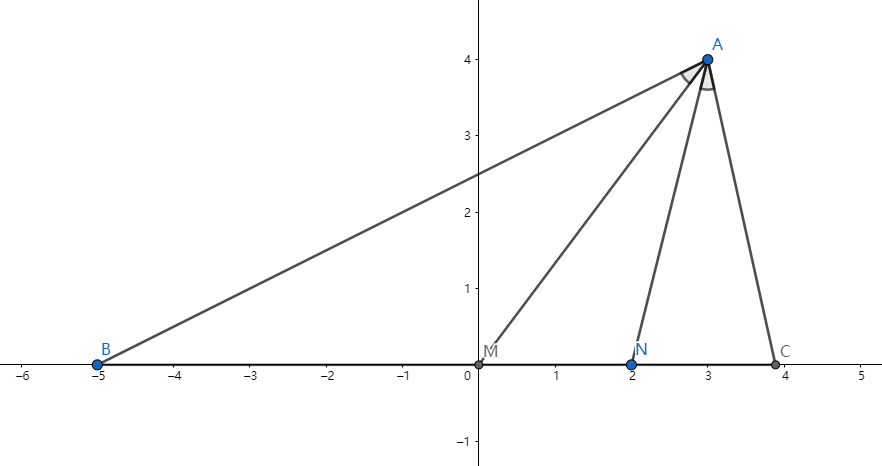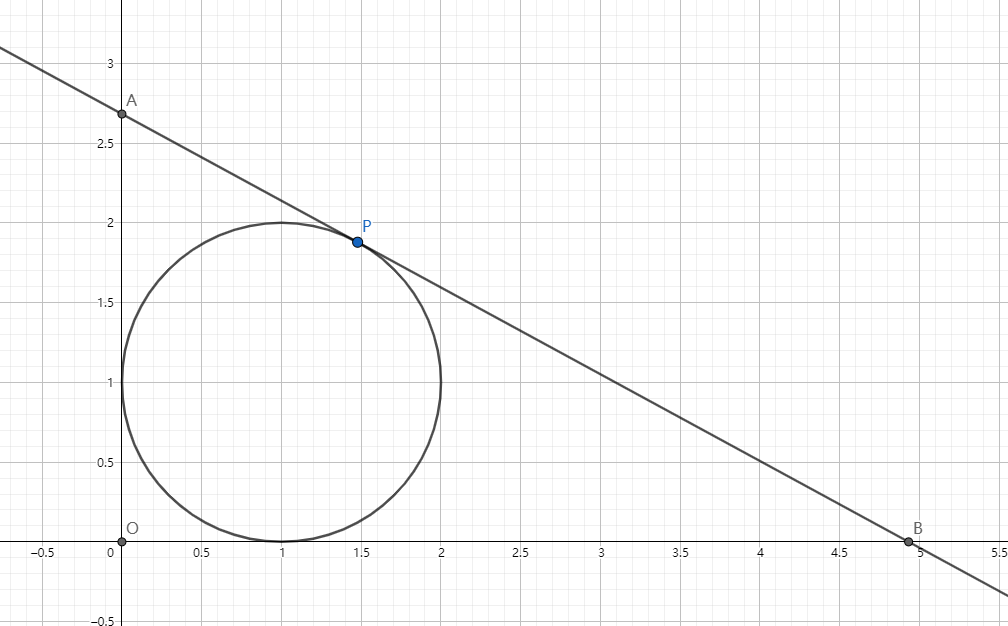# Reach for the Summit problem set - Mathematics

Welcome to the road to Olympics! By this problem set, you will lead your way to the summit of mathematics, just like climbing mountain in Celeste.

To match the Celeste scheme, each section will be seperated into A,B,C sides, each of which has lots of problems, having the similar format. The difficulty of the problems may be randomly shuffled, though.

I'll use this problem set for my games or programs later on, and I will upgrade it frequently.

Problem Format: For example, if the problem is on Stage 1, A side and 5th position, then the name of the problem will be:

Reach for the Summit - M-S1-A5

Stage 1:

• Sets and PIE

• Concepts, Properties, Graphs of Functions

• Derivatives and Extrema

Stage 2:

• Trigonometric Functions

• Trigonometric Identities

Stage 3:

• Sequences

Stage 4:

• Complex Numbers

Stage 5:

• Euclidean Geometry

Stage 6:

• Lines

Stage 7:

• Solid Geometry

• Vectors

Stage 8:

• Inequalities

• Geometric Inequalities

Stage 9:

• Functional Equations

• Polynomials

Stage 10:

• Combinatorics

• Combinatorics and Counting

• Combinatoric Identities

• Probability

Stage 11:

• Divisibility

• Modular Arithmetic

Stage 12:

• Number Bases

• Floor and Ceiling Functions

• Diophantine Equations

Stage 13:

• Integer Points

Stage 14:

• Coloring Problems

Stage 15:

• Existence Problems

• Combinatoric Geometry

Stage 16:

• Graph TheoryNote by Alice Smith
1 year ago

This discussion board is a place to discuss our Daily Challenges and the math and science related to those challenges. Explanations are more than just a solution — they should explain the steps and thinking strategies that you used to obtain the solution. Comments should further the discussion of math and science.

When posting on Brilliant:

• Use the emojis to react to an explanation, whether you're congratulating a job well done , or just really confused .
• Ask specific questions about the challenge or the steps in somebody's explanation. Well-posed questions can add a lot to the discussion, but posting "I don't understand!" doesn't help anyone.
• Try to contribute something new to the discussion, whether it is an extension, generalization or other idea related to the challenge.

MarkdownAppears as
*italics* or _italics_ italics
**bold** or __bold__ bold
- bulleted- list
• bulleted
• list
1. numbered2. list
1. numbered
2. list
Note: you must add a full line of space before and after lists for them to show up correctly
paragraph 1paragraph 2

paragraph 1

paragraph 2

[example link](https://brilliant.org)example link
> This is a quote
This is a quote
    # I indented these lines
# 4 spaces, and now they show
# up as a code block.

print "hello world"
# I indented these lines
# 4 spaces, and now they show
# up as a code block.

print "hello world"
MathAppears as
Remember to wrap math in $$ ... $$ or $ ... $ to ensure proper formatting.
2 \times 3 $2 \times 3$
2^{34} $2^{34}$
a_{i-1} $a_{i-1}$
\frac{2}{3} $\frac{2}{3}$
\sqrt{2} $\sqrt{2}$
\sum_{i=1}^3 $\sum_{i=1}^3$
\sin \theta $\sin \theta$
\boxed{123} $\boxed{123}$

Sort by:

Let set $A=\{x|x=a^2+b^2, a,b \in \mathbb Z\}$.

If $x_1,x_2 \in A$, is it always true that $x_1 \cdot x_2 \in A$?

- 1 year ago

Given that $a,b \in \mathbb R,\ a>0$, if $4^a-3a^b=16,\ \log_{2}a=\dfrac{a+1}{b}$, find the sum of all possible value(s) of $a^b$.

- 1 year ago

Find the monotonic increasing interval for function $y=\log_{0.5}(x^2+4x+4)$.

- 1 year ago

If $a>0,\ f(x)=\sqrt{x}-\ln(x+a)$ is monotonic increasing for $x \in (0,+\infty)$, find the minimum value of $a$.

- 1 year ago

Compare $\cos(\sin x)$ and $\sin(\cos x)$ for $x \in [0,\pi]$.

- 1 year ago

Without using the calculator, find the value of:

$(\sec 50 \degree + \tan 10 \degree)(\cos \dfrac{2 \pi}{7}+\cos \dfrac{4 \pi}{7}+ \cos \dfrac{6 \pi}{7})(\tan 6 \degree \tan 42 \degree \tan 66 \degree \tan 78 \degree)$

Let $A$ denote the value. Submit $\lfloor 10000A \rfloor$.

- 1 year ago

Given a positive infinite sequence $\{a_n\}$, $S_n = \displaystyle \sum_{k=1}^{n} a_k$.

If $\forall n \in \mathbb N^+$, the arithmetic mean of $a_n$ and $2$ is equal to the geometric mean of $S_n$ and $2$, then find $a_{2020}$.

- 1 year ago

In sequence $\{a_n\}$, $a_1=1,a_2=3,a_3=6$, for $n \geq 4,\ a_n=3a_{n-1}-a_{n-2}-2a_{n-3}$.

Then $\forall n \geq 4$, $a_n>\lambda \times 2^{n-2}\ (\lambda \in \mathbb N^+)$, then find the maximum value of $\lambda$.

- 1 year ago

If $z$ is one of the $7^{th}$ roots of unity, $z \neq 1$, find $(|z+z^2+z^4|)^2$.

- 1 year ago

If three complex roots of the equation: $x^3+px+1=0$ form an equilateral triangle on the complex plane, then find the area of that triangle.

Let $S$ be the area. Submit $\lfloor 1000S \rfloor$.

- 1 year ago

Find the value of:

$\displaystyle (\sum_{k=0}^{1010} (-1)^k {2020 \choose 2k} )^2 + (\sum_{k=0}^{1009} (-1)^k {2020 \choose 2k+1} )^2 \pmod {998244353}$

- 1 year agoAs shown above, in $\triangle ABC$, $A(3,4), B(-5,0), N(2,0)$, $C$ is a point on the positive x-axis such that $\angle BAM = \angle CAN$.

If the circumcenter of $\triangle AMN$ is $D(x_1,y_1)$, the circumcenter of $\triangle ABC$ is $E(x_2,y_2)$,

Find $\lfloor 1000(x_1+2y_1+3x_2+4y_2)\rfloor$.

Hint: Find the relationship of $AD$ and $AE$.

- 1 year ago

Find the smallest and largest inscribed equilateral triangle in an $1 \times 1$ square.

If the smallest triangle has area $S_{min}$, the largest has area $S_{max}$, submit $\lfloor 10^6 (S_{max}-S_{min})\rfloor$.

- 1 year agoAs shown above, a copied version of this image of Alice has been scaled uniformly, rotated and put inside the original one.

Then how many points are there correspond to the same relative position of the copy and the original one?

- 1 year ago

Given that $A(1,-1), B(-4,5)$, $C$ is on line $AB$, and $|AC|=3|AB|$, then find all possible coordinates of point $C$.

How to submit:

• First, find the number of all possible solutions $(x,y)$. Let $N$ denote the number of solutions.
• Then sort the solutions by $x$ from smallest to largest, if $x$ is the same, then sort by $y$ from smallest to largest.
• Let the sorted solutions be: $(x_1,y_1), (x_2,y_2), (x_3,y_3), \cdots ,(x_N,y_N)$, then $M=\displaystyle \sum_{k=1}^N k(x_k+y_k)$.

For instance, if the solution is $(-1,2), (-1,1), (1,3), (0,4)$, the sorted solution will be: $(-1,1), (-1,2), (0,4), (1,3)$, then $N=4$ and $\\ M=\displaystyle \sum_{k=1}^4 k(x_k+y_k)= 1 \times (-1+1) + 2 \times (-1+2) + 3 \times (0+4) + 4 \times (1+3) =30$.

For this problem, submit $\lfloor M+N \rfloor$.

- 1 year agoAs shown above, line $l$ is tangent to curve $C:x^2+y^2-2x-2y+1=0$ and it intersects with y-axis at point $A$, x-axis at point $B$, $O$ is the origin, $|OA|>2, |OB|>2$. Then find the minimum area for $\triangle AOB$.

Let $S$ denote the minimum area. Submit $\lfloor 1000S \rfloor$.

- 1 year ago

If point $A,B,C,D$ are in the $3D$ space, $\angle ABC = \angle BCD = \angle CDA = \angle DAB = 90 \degree$, is it always true that $A,B,C,D$ are on the same plane?

- 1 year ago